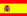﻿ Online calculator - Add or Subtract a PercentageYou are here : > Home> Utilities > Mathematics & Statistics > Add or Subtract a Percentage >

### Add or Subtract a Percentage

To calculate a total added or subtracted by a percentage, enter data as requested. Enter the percentage with negative sign if the value is to decrease the total. Use the point as decimal separator. Example, for 2,533.34 enter: 2533.34; The result is showed, automatically by clicking on "Calculate."

 Add or Subtract a Percentage Decimal places: Number: A % to add or subtract: Value (added / subtracted): Accumulated total